Welcome to Shenzhen Power Motor Industrial Co., Ltd. official website!
• English
• | Skype：info.power@power-motor.com

### Optimization analysis of brushless motor in cooking machine products

1. A basic overview of cooking machine products

Cooking machine, also known as the smart cooking machine, cooking can be done machines, cookers, rice cookers, steamers, and other comprehensive cooking, soybean milk, yogurt, juice and other special cooking machines, meat grinder, beat eggs and other food processing, so the cooking machine has the reputation of "world gourmet automatic cooking expert", which is more in line with modern people's needs for improving the quality of life.

As the power mechanism of the cooking machine, the motor drives the scraper and agitator to stir or chop food. Most of the products on the market use the direct drive. Under working conditions such as kneading, the motor speed is low but the required torque is large, otherwise, the motor will be blocked; under working conditions such as juice, the motor needs to have a high speed to prevent insufficient food granularity. The cooking machines currently on the market generally use switched reluctance motors. Switched reluctance motor is a new type of speed regulating motor, which has the advantages of large starting torque and wide speed range. At low speed, the controller outputs less current and the motor gets a larger starting torque. When the starting torque reaches 150% of the rated torque, the starting current is only 20% of the rated current; at high speeds, the motor realizes a wide speed adjustment range. However, the shortcomings of the switched reluctance motor are also obvious. The torque generated on the rotor of the switched reluctance motor is superimposed by impulse torque, so the torque pulsation and noise vibration are large.

A Brushless motor (BLDC) is developed based on a brushed DC motor. It overcomes the congenital defects of brushed DC motor, replaces mechanical commutator with an electronic commutator. Because the rotor adopts permanent magnets, the power density High, the same power motor is smaller, and the rated current can output the rated torque at low speed. The Brushless motor (BLDC) divided into square wave drive and sine wave drive according to the driving waveform, the sine wave drive can improve the motor running effect and make the output torque uniform and reduce the noise. Therefore, the brushless motor (BLDC) can sample the sine wave drive To solve the noise problem of switched reluctance drive, the output torque capacity and high-speed speed regulation of the brushless motor (BLDC) at low speed need to be analyzed and optimized.

2. Market demand and pain points

The requirements and pain points of the cooking machine for the motor are summarized as the following three points:

First, Low-speed and high-torque requirements, the motor torque needs to be low-speed kneading around 3N, considering that the torque demand for hard food may reach 5N.

Second, Wide speed range requirements, cooking machines need to complete a variety of special cooking requirements, so the motor needs to work in a wide speed range, and the rotation speed is required to be between 50 and 10,000 RPM.

Third, Household products need to solve the noise problem of products.

3. Optimization analysis of the solution

3.1 Analysis of the original solution

To solve the motor mute problem, the cooking machine uses a brushless motor sine wave drive to solve the motor noise problem. The following analyzes whether the torque of the first version of the motor solution meets the requirements, the motor line resistance R=1 ohm, the d-axis inductance Ld =0.0017H, q-axis inductance Lq=0.0027H, motor back EMF Ke=13.9V/k rpm.

Motor torque constant: Kt=0.0165×Ke=0.229N.m/A

In the controller control mode with id=0, output torque: T=Kt×I=0.23×I

When the output torque T=3N, the corresponding output phase current RMS=13A. if the maximum output torque is 5N, the corresponding output phase current RMS=21.7A, When the actual motor is testing the continuous current, the motor will heat up and burn the motor when the effective value of the current is 10A, so the torque constant of the prototype is too small and needs to be optimized.

3.2 Torque constant optimization

In the control mode with id=0, the torque constant is defined as the ratio of torque to current. Therefore, to achieve the large torque required by the product at low speed, the motor must be designed with a larger torque constant to achieve the same torque output. The current is smaller. To verify the effect of the torque constant optimization, the number of turns of the stator winding of the prototype is increased from 30 turns to 100 turns. At this time, the parameters of the optimized motor are as follows:

Motor wire resistance R=6.8 ohms, d-axis inductance Ld=0.0147H, q-axis inductance Lq=0.0247H, motor back EMF Ke=38.6V/krpm.

Motor torque constant: Kt=0.0165×Ke=0.63N.m/A

In the controller control mode with id=0, output torque: T=Kt×I=0.63×I

In the actual test, the effective value of the output current of the controller is about 5A, and the output torque is about 3N. Whether the torque constant is appropriate, it is necessary to comprehensively consider that the actual cooking machine’s maximum continuous torque demand of the motor is less than the actual rate. Prevent the continuous output power from causing the motor to heat up and burn the motor seriously.

3.3 Limitation of motor speed to torque coefficient

The torque coefficient increases. For the same torque, the output current will be smaller. Can the torque coefficient be arbitrarily enhanced to solve the torque output problem of the motor? The answer is no.

Due to Kt=0.0165×Ke, the increase of the torque coefficient will cause the increase of the back-EMF coefficient, and the increase of the back-EMF coefficient will directly cause the maximum speed of the motor to decrease. The maximum output voltage of the motor controller under SVPWM modulation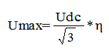Among them, Udc is the controller bus voltage=310V, generally 0.95, after calculation Umax=170V.

Without special processing by the control algorithm, Ke=13.9 V/krpm of the original prototype, and the maximum theoretical speed of the prototype=Umax/Ke=12230 rpm. After increasing the number of turns, the prototype Ke=38.6 V/krpm, and the maximum theoretical speed of the prototype =Umax/Ke=4404 rpm.

After calculation and analysis, increasing the torque constant can solve the torque output problem of the motor at low speed, but the increase of the torque constant will cause the maximum speed of the motor without special algorithm processing to decrease, for example, when the number of turns of the motor is increased, the maximum motor speed The theoretical speed drops to about 4400 rpm, so it is necessary to use the field weakening algorithm to extend the speed range of the motor.

Field weakening control is currently a research hotspot of PMSM. Motors can achieve high-speed operation by weakening the magnetic field (torque is also reduced) to expand the maximum speed. For PMSM, because the rotor is a permanent magnet, the field weakening control cannot be achieved simply by controlling the excitation current. An excitation current can be applied in the direction that offsets the permanent magnet flux to achieve field weakening control. The motor cannot be infinite when the field is weakened. Extend the field weakening speed range, the specific field weakening limit needs to take into account the specific parameters of the motor. The specific field weakening analysis will be discussed later. Generally, the field weakening range is within 3 times the field weakening. In this case, the field weakening range is reduced as much as possible. When the number of turns of the motor is increased, the motor speed will be about 2.3 times the field weakening from 4400 rpm to 10,000 rpm required by the product.

3.4 Inductance parameter optimization

3.4.1 Introduction to MTPA Algorithm

Through the above analysis, the torque constant cannot be increased indefinitely. The increase of the torque constant will result in the decrease of the motor speed, and the requirement of the field weakening expansion speed will increase. Therefore, the torque constant should be increased appropriately. When the torque constant is appropriate, The motor can increase the torque output capacity of the motor through the MTPA algorithm.

When verifying the torque constant optimization, the motor d-axis inductance Ld=0.0147H, q-axis inductance Lq=0.0247H, you can see that the motor has a salient pole effect. For the salient pole motor control algorithm, you can use MTPA (maximum torque current ratio control). ) The algorithm reduces the current of the motor.

Motor torque formula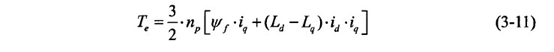Traditional vector control includes Id=0 control and maximum torque current ratio control. Since the DQ axis inductance of the surface mount PMSM is the same, the reluctance torque is not included in the torque expression, while for the built-in PMSM, Its DQ axis inductance is not equal, a part of the reluctance torque will appear in the torque expression, so through a certain control method, the reluctance torque can be used to minimize the stator current when outputting the same torque, and then Reduce losses and improve the efficiency of the motor, so the key point is to discuss which control method is used to achieve the same torque while minimizing the current.

Current limitation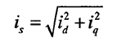To find the extreme value relationship, use the Lagrangian theorem in mathematics and introduce auxiliary functions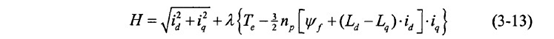Then began the process of Lagrangian extremum,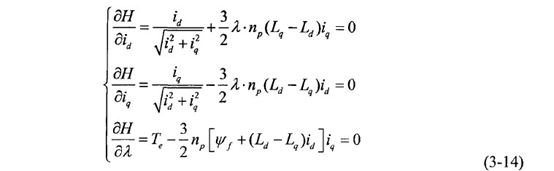The final id and it can be obtained respectively,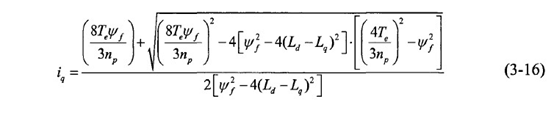3.4.2 Inductance parameter optimization direction

MATLAB is a scientific computing language and programming environment for algorithm development, data visualization, data analysis, and numerical calculation. Using Matlab tools can simulate motor control algorithms. Use the permanent magnet motor parameters with modified turns for simulation calculation, and you need to pay attention to the flux linkage parameters

Phyf=2/3×Pn×Kt=0.108Wb

The simulation result in the figure below shows that the effective value of the input current is 7A, and the maximum output torque Te=5.2Nm is calculated by the MTPA algorithm.

If the control strategy of Id=0 is adopted, the effective value of input current is required for the same torque Te=5.2N=Te/Kt=8.2A. That is, the same torque adopts the MTPA algorithm and the Id=0 control algorithm, and the current difference is 1.2A.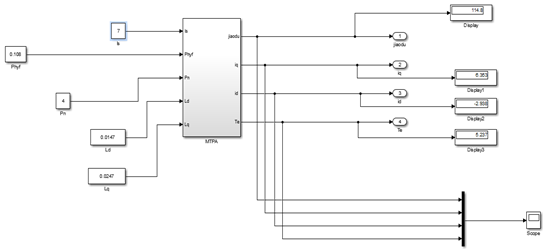The MTPA algorithm can use part of the reluctance torque, so the brushless motor can use the algorithm to reduce the output current of the same torque. According to the torque formula, it can be seen that the size of the reluctance torque and (Ld-Lq) The difference is related to the magnitude of the difference, so to better exert the reluctance torque of the brushless motor, the difference of (Ld-Lq) needs to be relatively large.

4. Through the above analysis, the optimization points of the motor can be obtained as follows:

First, the torque coefficient of the trial prototype is relatively small. To achieve the torque demand at low speed, the torque coefficient of the motor needs to be increased.

Second, the torque coefficient of the prototype cannot be increased indefinitely. The field weakening capability of the motor needs to be taken into consideration. The field weakening expansion speed of the motor needs to be within 3 times, which is as small as possible while meeting the torque output capability.

Third, the torque coefficient cannot be increased indefinitely. The Ld and Lq inductance values of the motor can be adjusted to increase the saliency of the motor to facilitate the MTPA algorithm so that the motor can use part of the reluctance torque to improve the torque output capacity of the motor

From the control algorithm of the motor controller, the optimization directions are:

First, perform the MTPA algorithm at low speed to exert the maximum torque of the motor.

Second, after reaching the maximum theoretical speed of the motor, the field weakening algorithm needs to be carried out to extend the speed range of the motor.

## Products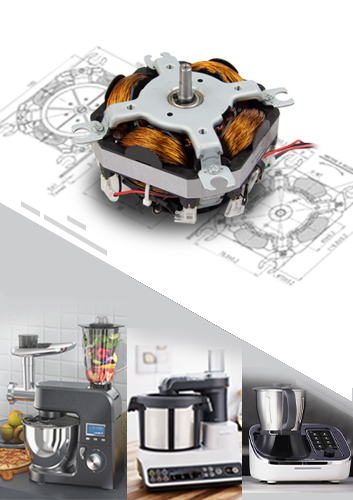## High efficiency flat motor PU1215 series for Cooking Machine or Kitchen Appliances, four-pole universal AC motor

##### Model:PU1215 series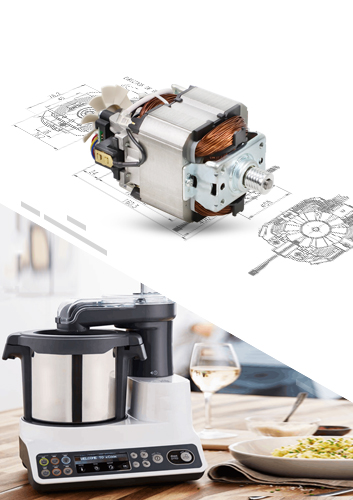## Mature kitchen machine motor 230V AC universal motor PU9540 series

##### Model:PU9540 series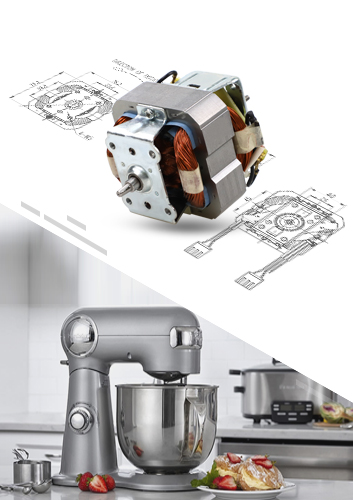## Mature kitchen machine motor 230V AC universal motor PU9525 series

##### Model:PU9525 series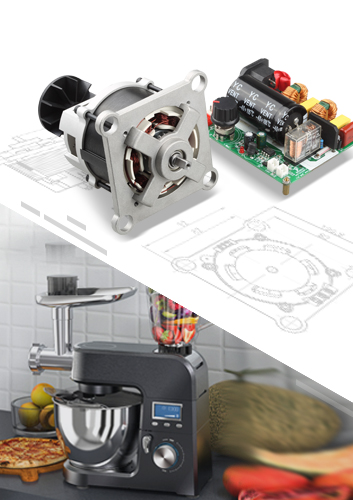## Kitchen Robot Brushless DC Motor Multi-function cook machine BLDC Motor

##### Model:PBL7640 series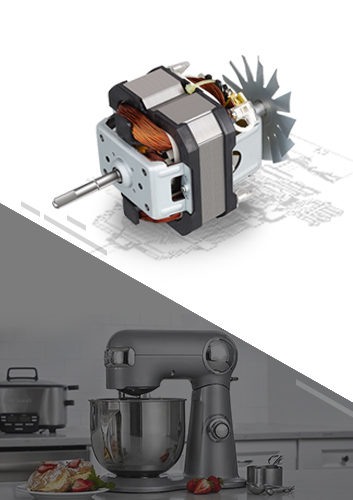## Kitchen Robot AC Universal Motor

##### Model:PU9828230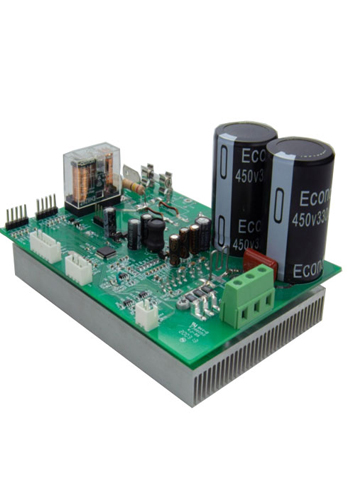## High voltage 3 phase brushless DC motor controller for cooker machine

##### Model:716PCAPTH0020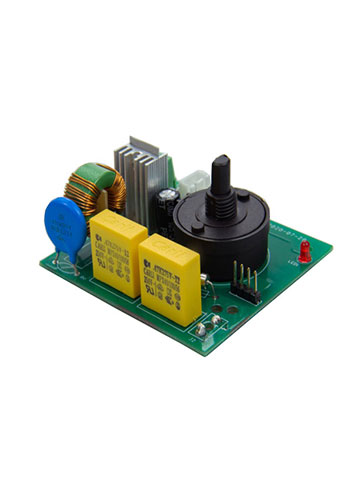## High voltage DC motor controller for cooker machine Multi-function mixer

##### Model:716PCDPTH0020

We use cookies and similar technologies on this website ("Cookies"). In order to allow their use to analyze website usage and to enhance functionality, please click on "Use all functions". To change the settings and select which specific Cookies we are allowed to use, or to obtain more detailed information, please click on "Settings".

Below, you can activate/deactivate the individual technologies that are used on this website.

Power Motor